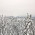## Wednesday, August 22, 2012

### How to interpret splits graphs

Splits graphs are produced by distance-based network methods such as NeighborNet and Split Decomposition, by character-based methods such as Median Networks and Parsimony Splits, and by tree-based methods such as Consensus Networks and SuperNetworks. They are all interpreted in the same way, which is discussed here.

An essential point to understand is that splits graphs are separation networks. That is, the edges in the graph represent separation between two clusters of nodes in the network; or, they split the graph in two. Formally, each edge represents a bipartition (or split) of the taxa based on one or more characteristics.

If there is no conflict in the data then each bipartition is represented by a single edge, and if there are contradictory patterns then the each bipartition is represented by a set of parallel edges. The edge lengths represent the relative amount of support in the whole dataset for each of the splits.

Example

As a simple example, I will use some data about opinion polls prior to a few Australian elections. There are data for nine election years: 1972, 1974, 1975, 1977, 1980, 1983, 1984, 1987, 1990. The data are for the actual winning margin as a result of the election, as well as data for various opinion polls predicting the outcome prior to the election: (i) McNair Survey, (ii) Roy Morgan Research, (iii) Saulwick Poll, and (iv) Other = pooling of Australian National Opinion Polls (data for 6 years), Spectrum (3 years), Newspoll (2 years), Levita (1 year).

I have calculated the Euclidean Distances between the results for the different opinion polls. So, the original data have been reduced to a set of distances between pairs of opinion polls; and it is these distances that are to be displayed by the network.

This is a simple dataset, and so the analyses based on Split Decomposition and NeighborNet turn out to be identical. The resulting network looks like this:

In this case, the network manages to represent all of the distances perfectly. That is, the Fit=100%. This is an improvement over trying to represent the data as a tree, instead. For example, the Neighbor Joining tree for these data has a fit of only Fit=92%, so that 8% of the information cannot be represented in the tree.

The network has five informative splits (bipartitions), each represented by a different set of parallel edges. The remaining five splits are simply shown as the single edges leading to each of the five sources of data. The informative bipartitions are (in order of decreasing support):
1. Actual Morgan Other      McNair Saulwick
2. Actual Morgan Saulwick   McNair Other
3. Actual Morgan            McNair Other Saulwick
4. Actual Other             McNair Morgan Saulwick
5. Actual McNair Other      Morgan Saulwick
These bipartitions are each highlighted below, with red representing one partition and blue the other. The weight of each split is also shown, which represents the amount of support there is in the data. This also determines the relative lengths of the edges (greater weight = more support = longer edge).

We can now start to reach some conclusions about the relative success of the opinion polls. For example, note that the three best-supported partitions (bipartitions 1, 2 and 3) associate Actual (the election result) with Morgan (the outcome predicted by Roy Morgan Research). We can thus conclude that this opinion poll has most in common with the election outcome, and thus that it was the most "successful" of the four opinion polls (over the elections from 1972 to 1990).

As noted, the edge lengths in the network represent the relative amount of support in the whole dataset for each of the splits. In this example, because Fit=100% the edge lengths along the shortest paths sum to exactly the original Euclidean Distances in the dataset, which will not always be so for other datasets. For example, the shortest distance from Actual to each of the four opinion polls is the sum of these edge lengths:
• Morgan   2.89 = 1.5833+0.4861+0.1875+0.6319
• Other    3.89 = 1.5833+0.4931+0.6493+1.1632
• McNair   4.25 = 1.5833+0.4931+0.6493+0.4861+0.8125+0.2257
• Saulwick 4.88 = 1.5833+0.4861+0.1875+0.4931+0.8125+1.3125
The calculation of the shortest distance from Actual to Saulwick is highlighted in this figure:

Note that there are several shortest paths from Actual to Saulwick — we can take the edges in any order we like so long as we cross each split only once. To go from Actual to Saulwick we have to cross four of the five informative splits, plus two of the other five splits.

Also worth noting is that the pathlengths in the Neighbor Joining tree do not sum to the Euclidean Distances. This is because the Fit<100%. For example, the pathlength from Actual to Saulwick is 4.74 = 1.8733+0.5278+0.6840+1.6539, so that 4.88–4.74 = 0.14 of the distance has been left out.

The pathlengths can also be used to evaluate the relative success of the opinion polls. That is, the network pathlength distance from Actual to Morgan is the shortest, which we can interpret as indicating that Roy Morgan Research was the most "successful" of the four opinion polls. That is, its predictions were the "least different" from the actual election results, across all of the elections.

Finally, there are features of the data that cannot be displayed in the network. The network is a summary only, and not all of the information can be summarized in a line graph! Perhaps the most notable missing information is that the McNair Survey was the only opinion poll to predict any of the election results exactly correctly, which it managed to do twice (in 1974 and 1983).

If you are interested in learning more about splits graphs, then you can check out the Primer of Phylogenetic Networks web page.

#### 1 comment:

1.Thanks for this nice and lucid explanation!